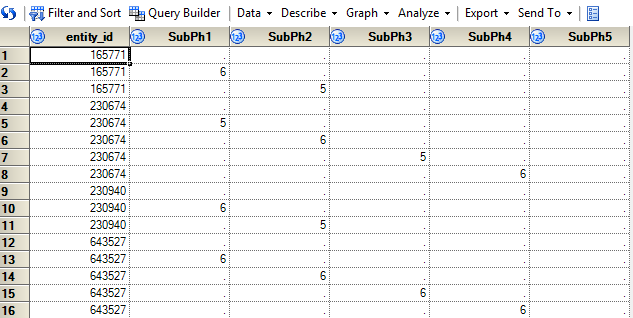## Collapse multiple records into one and combine column values

Hi Everyone

I would like to collapse the below records (screenshot) that have the same entity_id  into one record and keep all the column values, where one is populated.

Is there a fairly straight forward way of doing this?

Paul1 ACCEPTED SOLUTION

Accepted Solutions

## Re: Collapse multiple records into one and combine column values

Hi Paul,

Try this:

``````proc summary data=have nway;
class entity_id;
var SubPh:;
output out=want(drop=_:) max=;
run;``````
3 REPLIES 3

## Re: Collapse multiple records into one and combine column values

Hi Paul,

Try this:

``````proc summary data=have nway;
class entity_id;
var SubPh:;
output out=want(drop=_:) max=;
run;``````

## Re: Collapse multiple records into one and combine column values

Thanks a lot Freelance! That did it.

I have not used Proc Summary before. What do the below aspects of it do?

(drop=_:) max=

Paul

## Re: Collapse multiple records into one and combine column values

Maybe you have used (or heard of) PROC MEANS? It's almost the same, but writes to the output window by default.

The option MAX= of the OUTPUT statement says that

1. for each analysis variable specified in the VAR statement* the maximum is the summary statistic to be computed.
2. The names of the variables in the output dataset (here: WANT) containing the summary statistics shall be the names of the corresponding analysis variables (i.e., the maximum of SubPh1 shall be stored in a variable SubPh1, etc.). Otherwise, the new names would need to be listed after "MAX=".

By default, the output dataset contains variables _TYPE_ and _FREQ_ containing additional information about the summary: In your example, _TYPE_=1 for all observations, hence not very interesting, _FREQ_ = number of observations summarized, i.e. 3 for entity_id=165771, 5 for entity_id=230674, ... Assuming that you don't need these variables, I dropped them. More precisely: I dropped all variables whose names start with an underscore.

* Here, the VAR statement contains the list of all variables in dataset HAVE whose names start with "SubPh" (assuming that these are exactly your intended analysis variables).

Discussion stats
• 3 replies
• 1388 views
• 1 like
• 2 in conversation